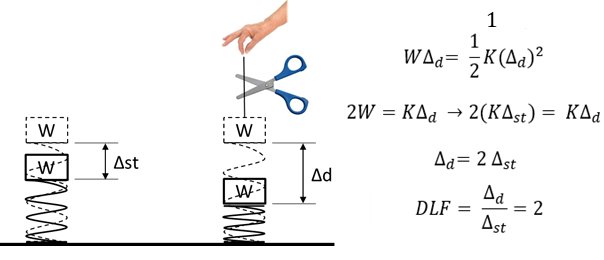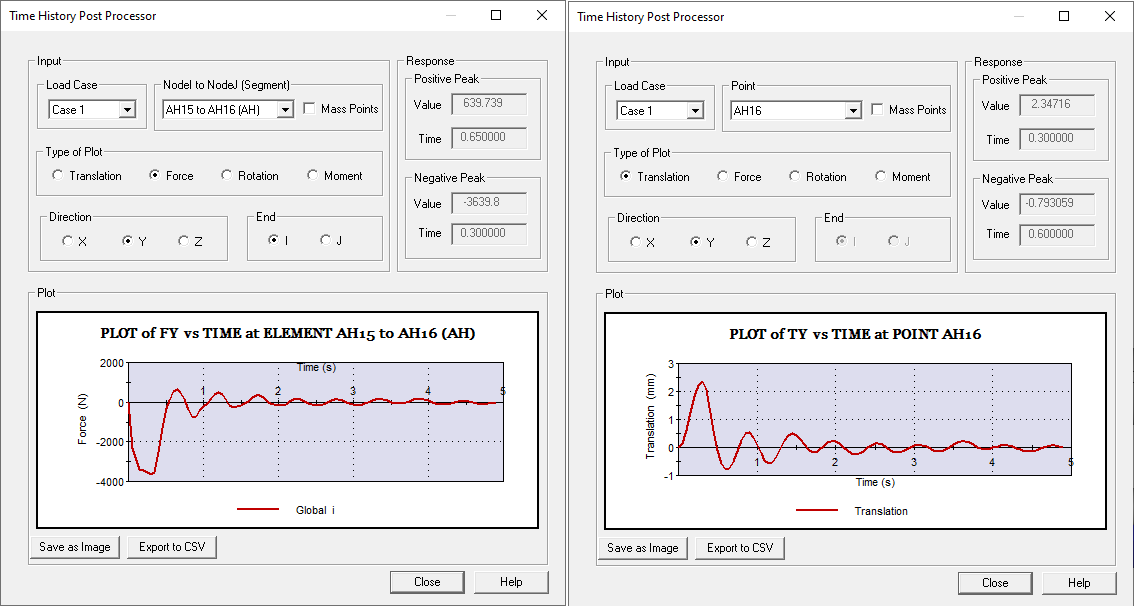# Time History Analysis

### Time History Analysis

A dynamic structural problem with a force function with a defined histogram can be solved using the approach of a small time step at a time. This small time step approach is called time history analysis.

The image on the left shows a force that varies over time, the force can be simulated as a series of rectangular forces with an equal but very short duration. This very short duration is what is called a time step.

Using this procedure, any structural dynamics problem can be reduced to a series of structural problems, one after the other, with constant rectangular forces.

At each step, an analysis is run, using the results of the previous step as initial conditions. Since analyzing a time step is roughly the same as performing a static analysis of the system, a 100-step time history analysis is equivalent to running 100 static analyzes.

### Analysis by Static Equivalent or by Time History

One way to solve the problem in a simpler way is to calculate the static equivalent of the load. This is calculating the DLF (Dynamic Load Factor) of the load, multiplying the load by the DLF and solving the problem statically. The DLF is a value that varies between 0 and 2, since it depends on the response time of the system Vs the change time of the load.

In many cases, given the difficulty of calculating the DLF, it is decided to multiply the peak value of the dynamic load by a DLF = 2, apply all the loads at the same time and solve the static problem.

This solution is usually conservative, except if the load causes resonance so DLF> 2 and does not take into account vibration problems.The calculation by Time History is more exact and adapts to any load duration or system response.

### Time History Analysis

AutoPIPE can calculate in a single run multiple cases per Time History, such as:

• Surge.
• Triggering of safety valves.
• Slug Flow.
• Pump stop.
• Pump start.
• Turbine trip.
• Seismic analysis by Ground Motion.

The Force Vs Time profiles can be imported from any fluid transient analysis program, such as the image on the left, which corresponds to the stoppage of some pumps in a desalination plant, or they can be generated by the program itself.

AutoPIPE allows to perform seismic analysis by Time History (Ground Motion). In this case we must have the complete system and AutoPIPE will move the floor. This method is recommended by the CFE of Mexico.

### AutoPIPE Fluid Transient

AutoPIPE integrates a Fluid Transients calculator that generates force profiles for integration by Time History. This generator is very useful for rapid valve closure phenomena, such as the tripping of a turbine, the closure of a HIPPS system, or any system where a block valve quickly closes the pipe.

The calculation of the pressure of the wave is done by Joukowski and based on the speed of the wave in the pipe AutoPIPE calculates the travel time between the elbows, the maximum imbalance of the force, the time it lasts and when it starts.

Based on these calculations, the program generates a force Vs time profile for each straight section and a file that indicates where and when said profile should be applied. Therefore, the user only has to indicate the integration time and if he wishes to apply the ZPA correction method.

AutoPIPE can also user generate the profiles for the tripping of steam safety valves, following the guidelines and cases of ASME B31.1.

### Numerical Integration Methods

The fundamental scheme of the step-by-step modal integration of time history is to assume a certain behavior within the integration interval, then based on this assumption and the data at the beginning of the interval, calculate the response at the end of it. Naturally, the assumptions of behavior within the interval greatly affect the response at the end of the interval. The integration schemes have been developed guided by the search for accuracy and stability. Currently, the most popular integration schemes are: Piecewise Exact and Wilson-θ, both included in AutoPIPE.

From the assumed acceleration distribution in the interval, the displacement and velocity at the end of the interval can be expressed in terms of the acceleration at the end of the interval. Step-by-step integration is done by substituting the acceleration, velocity, and displacement at the beginning of the interval into the equilibrium equation to obtain the acceleration at the end of the interval.

### AutoPIPE Results

AutoPIPE automatically generates the equations for stress verification according to the code used.

In addition to generating the maximum loads and displacements of each point due to the application of the dynamic load, the AutoPIPE has a post-processor that indicates:

• Displacements in each axis as a function of time. Indicating the maximum in each direction and when it occurs.
• Forces and moments in each axis as a function of time. Indicating the maximum in each direction and when it occurs.

This data is very useful to solve problems or to see how displacements, loads and stresses are reduced. For example, the graph indicates the oscillations of the displacement curve, and therefore the frequency of oscillation. If we reduce the natural vibration mode closest to that frequency, we will reduce displacements, loads and stresses.The curve on the left shows the load transmitted on the Y axis between two nodes. The one on the right shows the displacements in the Y axis. Both graphs show the maximum and minimum values and the scale shows the period of oscillation.